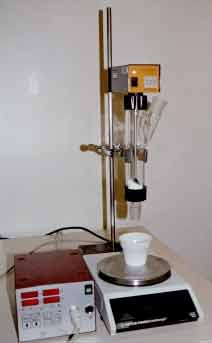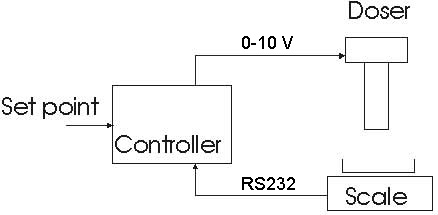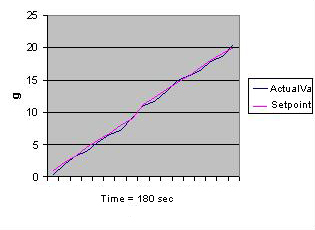Posted on

# Doser system for powder

We present here a set of devices wich connected together permit to add a defined amount of powder in a flask, fully automatically.

Material and methods

A PID controller ist connected to a balance trough a serial RS232 interface (fig. 1 and 2). The doser, wich contains the powder ist controlled by a 0-10 V signal. With 0 V the doser ist stopped, with 10 V it has maximal speed.figure 1 The devicesfigure 2. The connection between the devices
The user enter the amount of powder he want and the duration of the dosing. He press then the start button. The controller calculate at each time the amount of powder which should be added (set point). It compare this value with the weight measured at the scale, and control the speed of the doser.

This configuration has been used to add 3 time 5 g, and 3 time 20 g of NaCl powder. The controller parameter P=2 (proportional) and D = 0 (differential) have been used for this experiment.

Results

The results are reported in the table below. The figure 3 shows set point profile calculated from the controller (red) and the added powder (blue) in fonction time. On this curve you can see how the controller adjust the added amount to the calculated setpoint, wich follows a line.

 Set point (g) 5 5 5 20 20 20 Dosing time (min) 2 2 2 3 3 3 Powder amount measured (g) 4.8 5.2 5.3 20.4 20.1 20figure 3. Amount of powder added in function of time

Conclusion

The addition of powder with the doser has a good reproductibility , and a good precision (better then 0.5 g) The same construction can be made for liquid by replacing the doser with a peristaltic pump.

A new controller is in preparation. It will accept set point with 0.1 g precision.